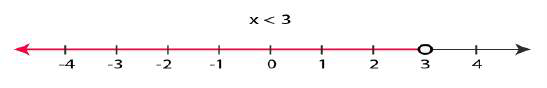# Important Questions for Class 11 Maths Chapter 6 - Linear Inequalities

Practice the Important questions for class 11 Maths Chapter 6 – Linear Inequalities, which are given here. In this chapter, students can learn how to derive the solution for a linear inequality, representation of the solution in the number line, and its graphical representation. Go through the article given below to get the important questions of class 11 chapter 6. Also, get all the chapters important questions for class 11 Maths here.

Class 11 Maths Chapter 6 – Linear inequalities comprise of the following important concepts:

• Inequalities
• The algebraic solution of a linear equation in one variable with its graphical representation
• Linear inequalities in two variables graphical solution
• Solution for the system of linear inequalities in two variables.

Also, Check:

## Class 11 Chapter 6 – Linear Inequalities Important Questions with Solutions

Practice and solve the important questions for class 11 Maths Chapter 6 – Linear Inequalities provided below.

Question 1:

Solve 3x+8>2, when

(i) x is an integer

(ii) x is a real number

Solution:

Given Linear inequality: 3x+8>2

The given inequality can also be written as:

3x+8 -8 > 2 -8 …(1)

In the above inequality, -8 is multiplied on both the sides, as it does not change the definition of the given expression.

Now, simplify the expression (1)

⇒ 3x > -6

Now, both the sides, divide it by 3

⇒ 3x/3 > -6/3

⇒ x > -2

(i) x is an integer

Hence, the integers greater than -2 are -1,0,1,2,…etc

Thus, when x is an integer, the solutions of the given inequality are -1,0,1,2,…

Hence, the solution set for the given linear inequality is {-1,0,1,2,…}

(ii) x is a real number

If x is a real number, the solutions of the given inequality are all the real numbers, which

are greater than 2.

Therefore, in the case of x is a real number, the solution set is (-2, ∞)

Question 2:

The cost and revenue functions of a product are given by C(x) = 20 x + 4000 and R(x) = 60x + 2000, respectively, where x is the number of items produced and sold. How many items must be sold to realise some profit?

Solution:

Given that,

Cost, C(x) = 20 x + 4000

Revenue, R(x) = 60x + 2000

We know that, profit = Revenue – Cost

Now, subsitute the given data in the above formula,

Profit = R(x) – C(x)

Profit = (60x + 2000)-(20 x + 4000)

Now, simplify it:

Profit = 60x + 2000 -20x -4000

Profit = 40x – 2000

To earn some profit, 40x – 2000 > 0

⇒40x > 2000

⇒ x>2000/40

⇒ x > 50

Thus, the manufacturer should sell more than 50 items to realise some profit.

Question 3:

Solve the given linear inequalities 3x-2 < 2x+1 and show the graph of the solution in the number line.

Solution:

Given linear inequality: 3x-2 < 2x+1

Bring the x terms on one side and constant terms on another side

⇒3x-2x < 1+2

⇒ x < 3

Therefore, the graphical representation for the solution of a linear inequality in number line is as follows:Question 4:

Ravi scored 70 and 75 marks in the first two-unit test. Calculate the minimum marks he should get in the third test to have an average of at least 60 marks.

Solution:

Assume that x be the marks obtained by Ravi in the third unit test.

It is given that the student should have an average of at least 60 marks.

From the given information, we can write the linear inequality as:

(70+75+x)/3 ≥ 60

Now, simplify the expression:

⇒ (145 +x) ≥ 180

⇒ x ≥ 180 -145

⇒ x ≥ 35

Hence, the student should obtain a minimum of 35 marks to have an average of at least 60 marks.

### Practice Problems for Class 11 Maths Chapter 6

Solve the given problems from class 11 Maths Chapter 6 – linear inequalities

1. A solution is to be kept between 40°C and 45°C. What is the range of temperature in degree Fahrenheit, if the conversion formula is F = (9/5)C +32?
2. Solve the given inequality for real x : (x/4)< [(5x-2)/3 -(7x-3)/5]
3. Solve the given inequality 3(1-x)<2(x+4) and represent the solution in the number line.
4. Determine all pairs of consecutive even positive integers, both of which are greater than 5 such that their sum is less than 23.
5. The longest side of a triangle is twice the shortest side and the third side is 2 cm longer than the shortest side. If the perimeter of the triangle is more than 166 cm then find the minimum length of the shortest side.
6. A solution is to be kept between 40°C and 45°C. What is the range of temperature in degree Fahrenheit, if the conversion formula is F= 9/5 C + 32?
7. Prove that the following system of linear inequalities has no solution:
x + 2y ≤ 3, 3x + 4y ≥ 12, where x ≥ 0, y ≥ 1
8. If x is real number and |x| < 3, then
(a) x ≥ 3
(b) -3 < x <3
(c) x ≤ -3
(d) -3 ≤ x ≤ 3

Download BYJU’S -The Learning App today and also learn all the Maths-related concepts with ease.